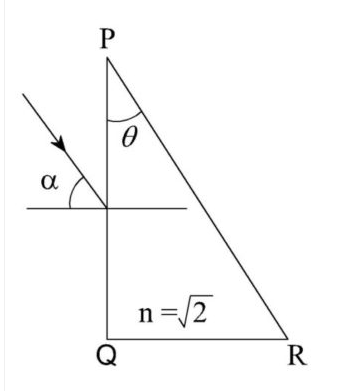User Name Remember Me? Password

 Advanced Optics Advanced Light and Optics Physics Help ForumOct 30th 2017, 11:47 AM #1 Junior Member   Join Date: Oct 2017 Posts: 1 Hard Optics Question A parallel beam of light is incident from air at an angle $a$ on the side $PQ$ of a right angled triangular prism of refractive index $n = \sqrt {2}$. Light undergoes total internal reflection in the prism at the face $PR$ when $a$ has a minimum value of $45°$. The angle $\theta$ of the prism is $...$ I'm so surprised for this question. May I get some help? Regards Here is my diagramJan 10th 2018, 07:57 AM #2 Junior Member   Join Date: Jan 2018 Posts: 2 Hey man, this is pretty straight forward, I'll try to lead you through. first calculate the minimal angle at which inner reflection happens (for a meterial with the given refraction index). Second, lets stick with an incident angle of alpha = 45°, since we know, that this is the angle, where inner reflection happens first at the surface PR. Calculate the angle after the surface PQ relative to the optical axis of PQ. Then transform this angle relative to the optical axis of Surface PR. This expression will contain the angle Theta. Now you can compare this expression with the above calculated angle, where inner reflection happens first. Thats it, let me know if you need further helpcheersTags hard, optics, questionThread ToolsShow Printable VersionEmail this Page Display ModesLinear ModeSwitch to Hybrid ModeSwitch to Threaded ModeSimilar Physics Forum Discussions Thread Thread Starter Forum Replies Last Post BearN Advanced Thermodynamics 0 Sep 22nd 2009 12:36 PM KaylaN Kinematics and Dynamics 1 Sep 22nd 2009 03:55 AM rjsci13 Kinematics and Dynamics 1 Sep 15th 2009 11:08 PM oblixps Kinematics and Dynamics 6 Sep 10th 2009 10:38 PM Inertialforce Advanced Electricity and Magnetism 1 Jan 7th 2009 05:20 PM